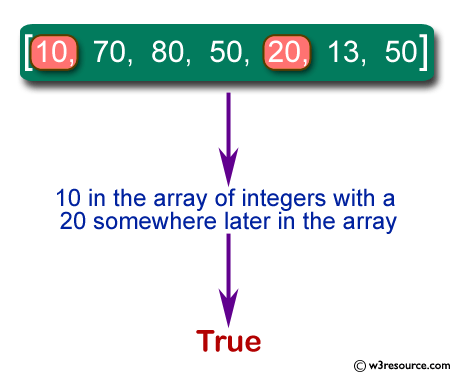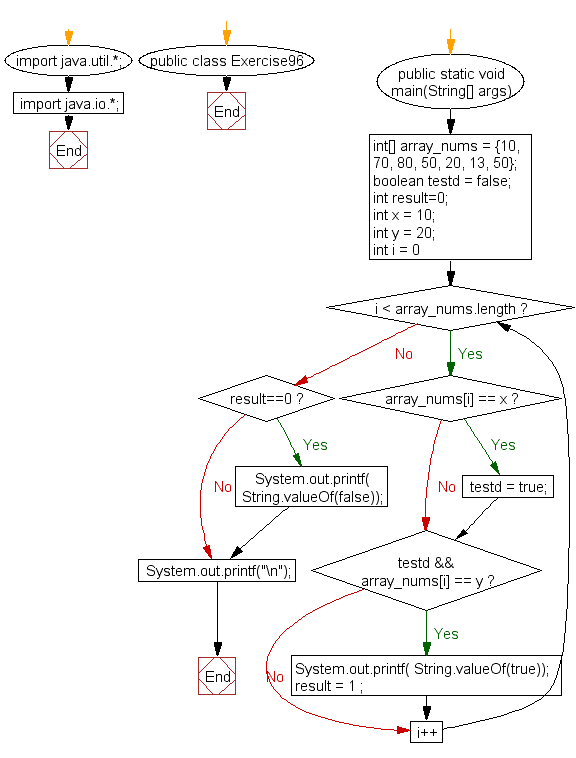﻿ Java exercises: Check if there is a 10 in a given array of integers with a 20 somewhere later in the array - w3resource# Java Exercises: Check if there is a 10 in a given array of integers with a 20 somewhere later in the array

## Java Basic: Exercise-96 with Solution

Write a Java program to check if there is a 10 in a given array of integers with a 20 somewhere later in the array.

Pictorial Presentation:Sample Solution:

Java Code:

``````import java.util.*;
import java.io.*;
public class Exercise96 {
public static void main(String[] args)
{
int[] array_nums = {10, 70, 80, 50, 20, 13, 50};
boolean testd = false;
int result=0;
int x = 10;
int y = 20;

for(int i = 0; i < array_nums.length; i++) {
if(array_nums[i] == x)
testd = true;

if(testd && array_nums[i] == y)
{
System.out.printf( String.valueOf(true));
result = 1		 ;
}
}
if (result==0)
{
System.out.printf( String.valueOf(false));
}
System.out.printf("\n");
}
}
```
```

Sample Output:

```true
```

Flowchart:Java Code Editor:

What is the difficulty level of this exercise?

﻿

## Java: Tips of the Day

Execution of console commands:

Java allows you to execute console commands directly from code using a class Runtime. It is very important not to forget about exception handling.

For example, let's try to open a PDF file through a Java terminal:

```import java.io.BufferedReader;
import java.io.InputStream;

public class ShellCommandExec {

public static void main(String[] args) {
String gnomeOpenCommand = "gnome-open //home//user//Documents//MyDoc.pdf";

try {
Runtime rt = Runtime.getRuntime();
Process processObj = rt.exec(gnomeOpenCommand);

InputStream stdin = processObj.getErrorStream();

String myoutput = "";